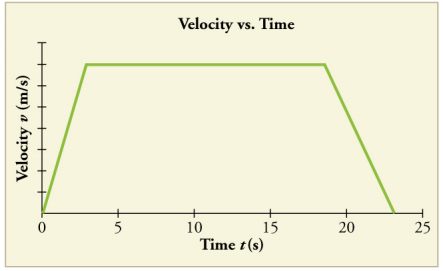# Problem: Consider the velocity vs. time graph of a person in an elevator shown in Figure 2.58. Suppose the elevator is initially at rest. It then accelerates for 3 seconds, maintains that velocity for 15 seconds, then decelerates for 5 seconds until it stops. The acceleration for the entire trip is not constant so we cannot use the equations of motion from Motion Equations for Constant Acceleration in One Dimension for the complete trip. (We could, however, use them in the three individual sections where acceleration is a constant.) Sketch graphs of (a) position vs. time and (b) acceleration vs. time for this trip.

###### FREE Expert Solution

Velocity is the rate of change of displacement of an object.

The area under the velocity vs. time graph gives the displacement of the object.

Acceleration is the rate of change of velocity of an object.

The slope of the velocity vs. time graph gives the acceleration of the object.

(a)

It is seen from the figure that the velocity of the person in the elevator increases up to 3 seconds.

The velocity is then maintained up to 18 seconds.

The velocity decreases up to 23 seconds.

86% (27 ratings)###### Problem Details

Consider the velocity vs. time graph of a person in an elevator shown in Figure 2.58. Suppose the elevator is initially at rest. It then accelerates for 3 seconds, maintains that velocity for 15 seconds, then decelerates for 5 seconds until it stops. The acceleration for the entire trip is not constant so we cannot use the equations of motion from Motion Equations for Constant Acceleration in One Dimension for the complete trip. (We could, however, use them in the three individual sections where acceleration is a constant.) Sketch graphs of (a) position vs. time and (b) acceleration vs. time for this trip.